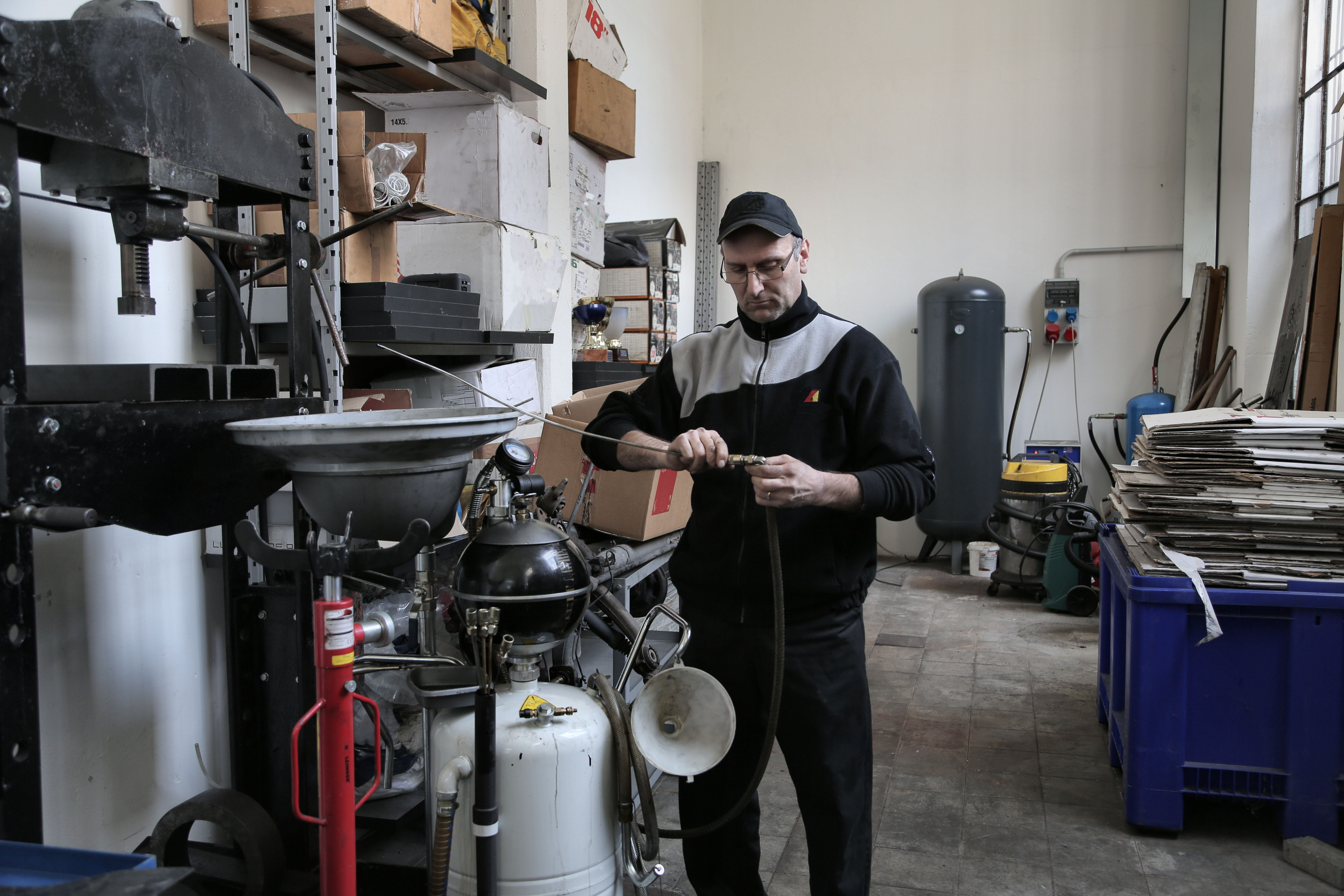# AC Challenge- Troubleshooting Simple Circuits 1 -197

Welcome to the ET 197 How to:
AC Challenge Troubleshooting Simple Circuits. We're at our AC Circuits Challenge
and we're gonna go straight to Troubleshooting Simple Circuits One
Let's begin . Let's hint.
So this is an AC circuit. It operates different than a DC
circuit. Let's go through the hints. A blown fuse is going to have a zero
current and our voltage at the bottom
of the fuse is going to measure zero volts. If we have a
capacitor that's open, we're going to get the full voltage
on both sides of this. If C1 is shorted, we're going to get a
non-zero current reading. Both sides of this terminal
are going to have the applied voltage. The other side we're
going to have the applied voltage. If it's shorted,
we're going to be the same on both sides. If our resistor is open we're going to get a voltage on top.
Which would be the applied voltage and we should get a
ground on the bottom. If R1 is shorted, we're going to get a non-zero rating.
They're will be current through there
and it'll be wrong.

We're going to get the same voltage any time that we
have a short. You're going to get the same voltage,
whenever you get a short. You're going to get the same voltage on both sides. So if it's open, we're not going to get
a complete circuit. We're going to get zero current if it's shorted. We're going to get a non-zero current and the bottom is going to be ground and the top should be the applied
voltage. If we have a trace open we're going
to measure on one side. If there are no faults, our capacitor
should be a higher voltage than our applied voltage.
That's because of the way the AC
circuits work. Resistors are fixed, so their AC and DC will be the

Capacitors under AC will be
different and inductors will be different. That's because of the complex
way that AC circuits work.
Okay enough talk, let's troubleshoot. Zero current means that we have an
open. So let's divide and conquer. 24 V – okay
and let's go down here. We got 24 and here we have 24.
Here we have ground, so that means probably our "L1",
which L's are our inductors, is open.

Good – Divide and conquer –
24Volts. 24 means, we have an open. No current
and voltage. Let's keep chasing it out 24, ground,
24. Well let's see here. That means our
Inductor is open again. All right! No current and full voltage.
We have an open somewhere. Oops- oops-okay. That means our resistor is open. We have voltage on one side and ground on the other side.
So let's say "R1" is open. Good!
Divide and conquer.Seven that is good. That is not good,
because here we have 18 volts on our capacitor. Okay-
Well in this case, our voltage is lower. That's probably okay. Let's go – nine volts.
Oh, we have a ground here. Not good and here not
good. I'm going to say our inductor is shorted. Good! We have current.
We have a higher voltage that means this guy is working good.
Let's go down here. We have no voltage drop
across our resistor. Let's go over here. Okay, I'm going to say our resistor.

Let's see Let's say our resistor is shorted. We
shouldn't be getting the same voltage on both sides. Good!
Divide and conquer. No current, we have an open. 24 volts okay.
Let's see what, We have a problem That's ground. That's ground.
That's ground. That's ground and that's a full voltage. So, I'm going to say Trace 2 is open. We
have voltage on one side and ground on the other, so I'm going to
say Trace 2 is open. Good ! Divide and conquer. Zero – No current- No voltage – No voltage – voltage.
So our Trace one. We have voltage on one side and nothing on the other. That
should be our Trace one – open. Good !
Again no current and no voltage.
We have voltage – no voltage, so it's open "C1" is open Again no current and same voltage.
We have an issue.

We just need to figure it out. What it is. Do we have a voltage drop across our? Nope- nope-nope. So
it looks to me like our Trace 4 is open. We have current and we have the same voltage on both
sides. That's a problem. Let's see. Do we get a voltage drop
across the resistor? Yes. So I'm going to assume our inductor is
okay. So it looks to me like the same voltage across. Looks like our capacitor is shorted Good! We are finished.
I'll show you again. Here just put your name in there and we're going to skip this disk
storage. Here that's okay. We're going to
print this to our Cute Pdf and upload it into
our Canvas..